# Environmental Health: Flow in a Waste Pipe and Trigonmetry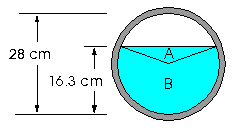A drainage pipe runs under the floor of a manufacturing facility. It is circular in cross-section with an inside diameter of 28 cm as shown to the right. By inserting a piece of doweling (a stick) in the drain in the floor, it has been determined that the depth of waste liquid in the pipe is 16.3 cm. If the rate at which the waste liquid is flowing to an underground waste facility is 0.78 m/s, determine how much water flows through the drainage pipe in Imperial gallons/minute.

## Solution:

The first step is to determine the cross-sectional filled area (shown in blue) by finding the area of the triangle, A, plus the area of the sector, B.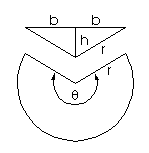The area of each triangle shown to the right is given by the formula: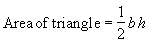The area of the sector can be found by multiplying the area of a circle: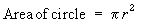by the fraction of a circle that the sector fills. If the sector’s angle is θ radians, then since a full circle is 2π radians, this area is: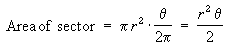Thus the total area is: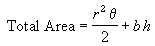The second step is to find r, h, b and θ from the information provided and evaluate this area.

• The radius is half the diameter, so r = 14 cm.
• From the radius and the depth it can be determined that h, the height of the two triangles is:
h = 16.3 - 14 = 2.3 cm.
• Using Pythagoras’ theorem we can find b: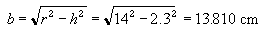• To determine θ, find the angle (call it A) between sides r and h in one of the triangles: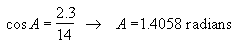and subtract: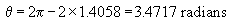Substituting all these values into the total area formula gives the total cross-sectional area of flow: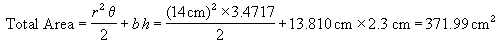Converting this to square meters gives: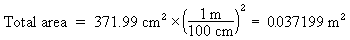The last step is to find the volume flow (the volume of liquid flowing through the pipe per unit time). This is given by the cross-sectional area times the velocity: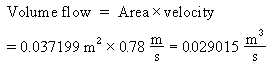All that remains now is to convert this result to the desired units, namely imperial gallons per minute: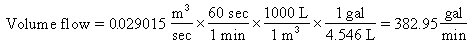Because the information presented in this problem was accurate to only 2 significant figures we must round our answer to 2 significant figures as well. Thus the flow rate of liquid waste through the pipe is 380 gal/min.Written by Louise Routledge, Thursday, December 12, 1996